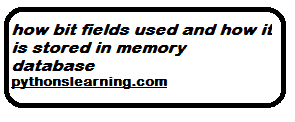## how bit fields used and how it is stored in memory database

In programming terminology, a bit field is a data structure that allows the programmer to allocate memory to structures and unions in bits in order to utilize computer memory in an efficient manner.

Here is a code in C that illustrates the implementation of a structured time without the use of bit fields:
CODE:how bit fields used and how it is stored in memory database
``````#include <stdio.h>
struct time
{
unsigned int hours;
unsigned int minutes;
unsigned int seconds;
};
int main()
{

struct time t = {11, 30, 10};
printf("The time is %d : %d : %d\n", t.hours, t.minutes, t.seconds);
printf("The size of time is %ld bytes.\n", sizeof(struct time));
return 0;
}

OUTPUT:
The time is 11: 30: 10
The size of time is 12 bytes.
``````

Clearly, we know that, for a 24-hour clock, the range of hours should be from 0 to 23, minutes, and seconds should be from 0 to 59.So, that we could apply bit memory concept on the above code and make it use less space.
In the above code the size is 12 bytes.

Here is a code in C that illustrates the use of bit-fields with the help of the previous example:

``````#include <stdio.h>
struct time
{
unsigned int hours: 5; // Size restricted to 5 bits
unsigned int minutes:6; // Size restricted to 6 bits
unsigned int seconds:6; // Size restricted to 6 bits
};
int main()
{

struct time t = {11, 30, 10}; // Here t is an object of the structure time
printf("The time is %d : %d : %d\n", t.hours, t.minutes, t.seconds);
printf("The size of time is %ld bytes.\n", sizeof(struct time));
return 0;
}
OUTPUT: